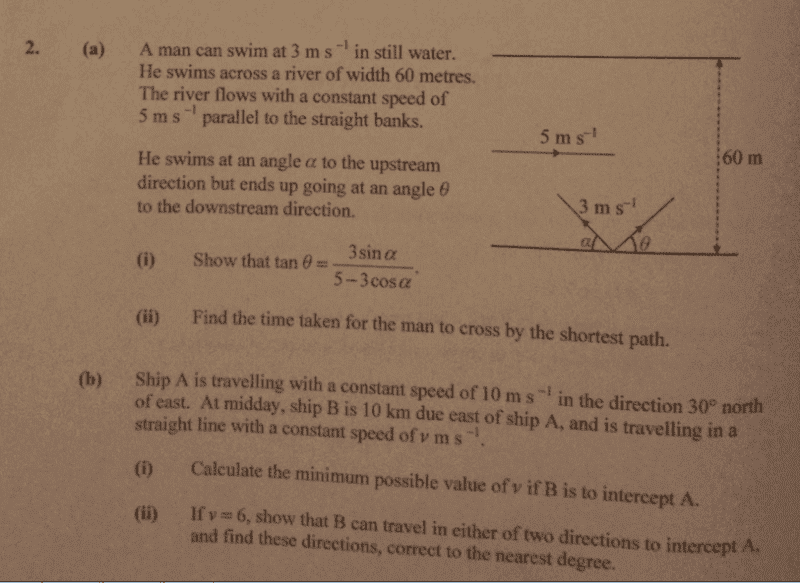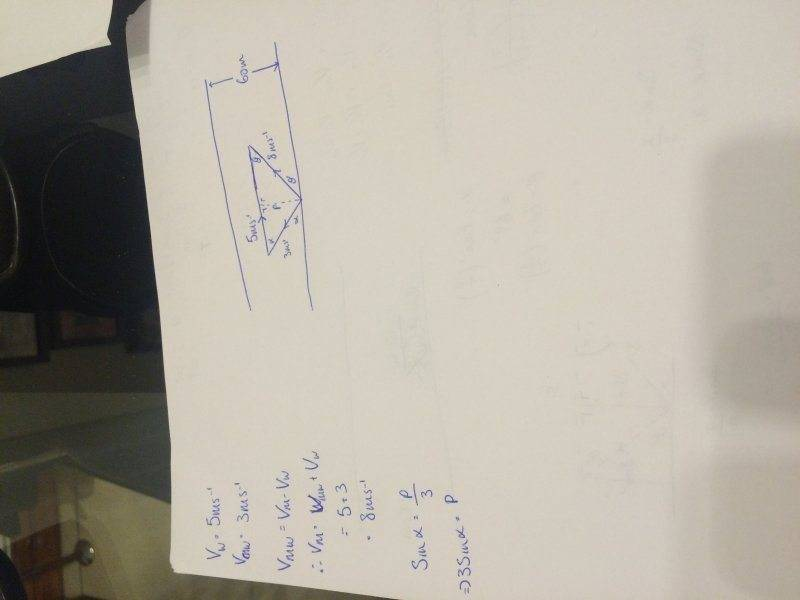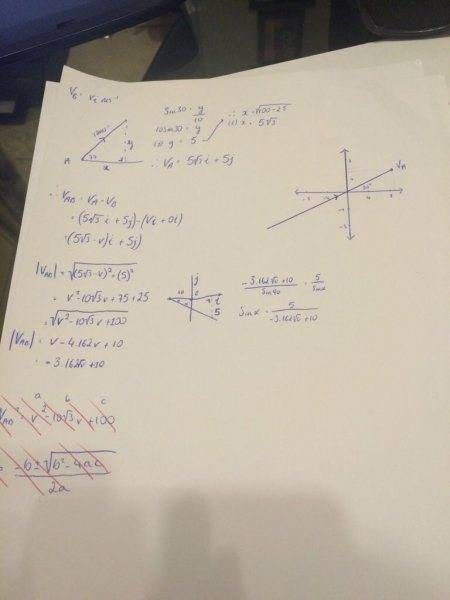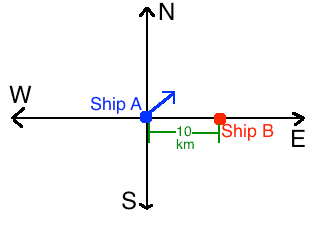# Relative Velocity; Help needed please

2. (a) is the question I'm stuck with## Homework Equations

I know that 3m/s is the Velocity of the man relative to the water and the Velocity of the man is 8m/s and is represented by the vector pointing in the direction theta downstream but I just can't get my head around this question, probably something simple that's alluding me! I have part (b) completed and it is meant to be the more difficult of the two.

## The Attempt at a Solution

I've attempted to add the vectors via triangle law but I can't seem to get it right my vector triangle always ends up having the hypotenuse as one of the shorter sides, any help would be appreciated!

Nathanael
Homework Helper
I know that ... the Velocity of the man is 8m/s

I've attempted to add the vectors via triangle

I just realised what I was doing wrong, but I'm still not finished the question, here's what I have so farNathanael
Homework Helper
You can only add the magnitude of vectors if they are in the same direction!! Add them component by component.

Here you go! :)

Thank you!

I'm a bit curious, how did you do b)? :)

•I like Serena
Nathanael
Homework Helper
Here you go! :)
It is good that you want to help, but you are not supposed to give full solutions. The point is for the OP to find the solution so that they can better understand.

It is good that you want to help, but you are not supposed to give full solutions. The point is for the OP to find the solution so that they can better understand.

I'm sorry Sir!

It is good that you want to help, but you are not supposed to give full solutions. The point is for the OP to find the solution so that they can better understand.
I prefer to be shown a solution and work it backwards to see how one arrives at that solution, I learn better that way!

Nathanael
Homework Helper
I prefer to be shown a solution and work it backwards to see how one arrives at that solution, I learn better that way!
When problems get harder, this method will break down. As a problem gets more complex, it gets increasingly difficult to understand other people's work. People have their own perspectives and their own ideas for solving things (there is very often many different ways to solve a problem) it's not always easy to understand what they were doing (even if you've solved the problem yourself!)
I'm glad you got something out of it, but if I may make a suggestion; I suggest trying to be as independent as possible when solving problems.

•Alettix and Aaron Curran
When problems get harder, this method will break down. As a problem gets more complex, it gets increasingly difficult to understand other people's work. People have their own perspectives and their own ideas for solving things (there is very often many different ways to solve a problem) it's not always easy to understand what they were doing (even if you've solved the problem yourself!)
I'm glad you got something out of it, but if I may make a suggestion; I suggest trying to be as independent as possible when solving problems.

turns out I didn't do part b correct, any pointers?

Nathanael
Homework Helper
turns out I didn't do part b correct, any pointers?
The shortest path is when θ is largest.
(Ideally we would want θ=90°, but this can't happen because even if the person swam directly against the current, they would still be moving downstream.)
Do you know how to find the maximum θ using calculus?

The shortest path is when θ is largest.
(Ideally we would want θ=90°, but this can't happen because even if the person swam directly against the current, they would still be moving downstream.)
Do you know how to find the maximum θ using calculus?

I mean the actual second part of the question about the ships moving in subsequent motion this is what I have so farNathanael
Homework Helper
I mean the actual second part of the question about the ships moving in subsequent motion this is what I have so far
Oh! Sorry, I'm a little blindI assume by "intercept" they mean something like "crash"Sorry I can't understand your thought process based off the image (I can't even read it very well). It would be helpful if you gave a brief explanation of your approach.

[[EDIT: Deleted my method, I didn't think it through; it is simpler than the method I proposed]]

Last edited:
The shortest path is when θ is largest.
(Ideally we would want θ=90°, but this can't happen because even if the person swam directly against the current, they would still be moving downstream.)

Do you know how to find the maximum θ using calculus?
Oh! Sorry, I'm a little blindI assume by "intercept" they mean something like "crash"Sorry I can't understand your thought process based off the image (I can't even read it very well). It would be helpful if you gave a brief explanation of your approach.

I haven't worked it out, but this is my first instinct for how to solve it:
I would find the speed it takes to crash as a function of the direction that ship B is traveling, and then I would find the minimum of this function.

It gives the velocity the ship is travelling and the direction in which it is travelling, so I broke this into i and j components, if given the velocity then the velocity vector will always be the hypotenuse of the triangle so using that and the angle by sin30 = y/10 then 10sin30 = y, which is 5 so 5j is one vector component of the ships movement and the other is 5root3 i and then I took the velocity of A relative to B which is the Velocity of A - Velocity of B so that was (5root3 - v)i + 5j and usually the find the magnitude of the relative velocity it would the square root of the i component squared + the j component squared but then I get an expression in V which is messy and I have no idea where to go from there.

also i is the horiztonal and j is the verticle component

Nathanael
Homework Helper
If the two boats crash, then they will have the same position at the same time.
Right now, the boats are at the same position along the North-South axis.
In the future, (when they crash) they will also have the same position along the North-South axis.
So what do you know about their North-South velocities?

If the two boats crash, then they will have the same position at the same time.
Right now, the boats are at the same position along the North-South axis.
In the future, (when they crash) they will also have the same position along the North-South axis.
So what do you know about their North-South velocities?

ahhh, the N/S velocity of A is 5j and the N/S velocity of B is 0j as it travels along the west-east axis

Nathanael
Homework Helper
ahhh, the N/S velocity of A is 5j and the N/S velocity of B is 0j as it travels along the west-east axis
But if B travels along the E/W axis, then will they ever crash?

Remember, the problem does not give a direction for boat B, you can make it whatever direction you want!

But if B travels along the E/W axis, then will they ever crash?

Remember, the problem does not give a direction for boat B, you can make it whatever direction you want!

as B travels along the E/W from east to west, A approaches from the south west (travelling E30degreesN and the problem is we have to find the minimum value of the i component of B's velocity for the two to intercept, and in the beginning B is 10km due east of A

Nathanael
Homework Helper
as B travels along the E/W from east to west, A approaches from the south west (travelling E30degreesN and the problem is we have to find the minimum value of the i component of B's velocity for the two to intercept, and in the beginning B is 10km due east of A
Where do you see that it says B travels along the E/W?
It seems to me that the problem is saying B can be traveling in any direction. I'm not sure why you keep saying it's traveling along the E/W axis

Where do you see that it says B travels along the E/W?
It seems to me that the problem is saying B can be traveling in any direction. I'm not sure why you keep saying it's traveling along the E/W axis

I actually don't know why I kept saying that, I'm still clueless as to what to do now though

Nathanael
Homework Helper
I actually don't know why I kept saying that, I'm still clueless as to what to do now though
Does the clue in post #17 not help?
If the two boats crash, then they will have the same position at the same time.
Right now, the boats are at the same position along the North-South axis.
In the future, (when they crash) they will also have the same position along the North-South axis.
So what do you know about their North-South velocities?

Does the clue in post #17 not help?

But the question states that Ship B is 10km due east of A when t=0 so they can't be in the same position along the N/S axis because that would mean that B would have to be either 10km due north or due south of A for this to be true?

Nathanael
Homework Helper
But the question states that Ship B is 10km due east of A when t=0 so they can't be in the same position along the N/S axis because that would mean that B would have to be either 10km due north or due south of A for this to be true?
"Position along the N/S axis" corresponds to what most might call the "y-coordinate" and it is the same for both ships:"Position along the N/S axis" corresponds to what most might call the "y-coordinate" and it is the same for both ships:
View attachment 78918

think i'm on the right track, will let you know if I get it solved, thanks for all your help so far!

"Position along the N/S axis" corresponds to what most might call the "y-coordinate" and it is the same for both ships:
View attachment 78918
Think I have it, the minimum value of the j (verticle/y component) is 5j (5km/h) and if the value of V=6 then the directions of interception are due north and N6.59degreesW

Nathanael
Homework Helper
Think I have it, the minimum value of the j (verticle/y component) is 5j (5km/h) and if the value of V=6 then the directions of interception are due north and N6.59degreesW
If it is going due north at 6 m/s it will go too far and not crash. You want the Northern component of velocity of B to be 5m/s then it will always eventually crash (with one exception) because they will always have the same N/S coordinate.

If it is going due north at 6 m/s it will go too far and not crash. You want the Northern component of velocity of B to be 5m/s then it will always eventually crash (with one exception) because they will always have the same N/S coordinate.

by intercept in the question I think they mean when ship B crosses the path which ship A is taking, in the case of a collision or going to take in the case of an interception? because 2.(b)(ii) asks that if v=6 show that B can travel in either of two directions to intercept A and find these directions.

The minimum value asked in part (i) is when they will collide but in part (ii) they are referring to the ships crossing paths I believe?

Nathanael
Homework Helper
by intercept in the question I think they mean when ship B crosses the path which ship A is taking, in the case of a collision or going to take in the case of an interception? because 2.(b)(ii) asks that if v=6 show that B can travel in either of two directions to intercept A and find these directions.

The minimum value asked in part (i) is when they will collide but in part (ii) they are referring to the ships crossing paths I believe?
I doubt it. If this is what they mean then there are infinite different directions which ship B could travel. But to crash, there are exactly 2 angles which it can travel.